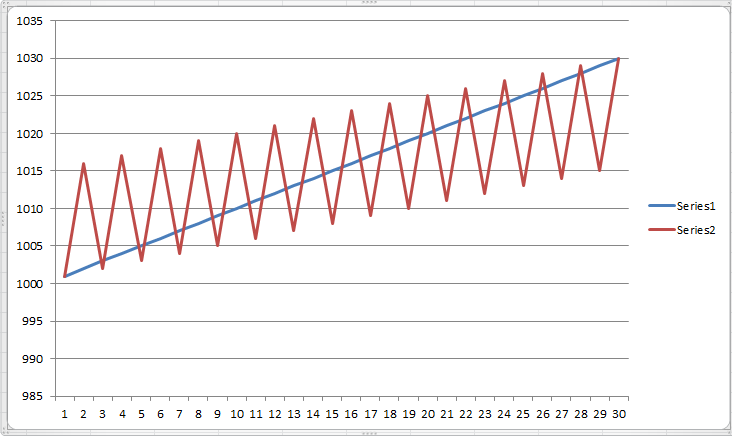## Volatility and price differentiation

In a rather surreal interview to the rather fantastically named Aurangzeb Naqshbandi and Hindustan Times editor Sukumar Ranganathan, Congress president Rahul Gandhi has made a stunning statement in the context of agricultural markets:

Markets are far more volatile in terms of rapid price differentiation, than they were before.

I find this sentence rather surreal, in that I don’t really know what Gandhi is talking about. As a markets guy and a quant, there is only one way in which I interpret this statement.

It is about how market volatility is calculated. While it might be standard to use standard deviation as a measure of market volatility, quants prefer to use a method called “quadratic variation” (when the market price movement follows a random walk, quadratic variation equals the variance).

To calculate quadratic variation, you take market returns at a succession of very small intervals, square these returns and then sum them up. And thinking about it mathematically, calculating returns at short time intervals is similar to taking the derivative of the price, and you can call it “price differentiation”.

So when Gandhi says “markets are far more volatile in terms of rapid price differentiation”, he is basically quoting the formula for quadratic variation – when the derivative of the price time series goes up, the market volatility increases by definition.

This is what you have, ladies and gentlemen – the president of the principal opposition party in India has quoted the formula that quants use for market volatility in an interview with a popular newspaper! Yet, some people continue to call him “pappu”.

## Why standard deviation is not a good measure of volatility

Most finance textbooks, at least the ones that are popular in Business Schools, use standard deviation as a measure of volatility of a stock price. In this post, we will examine why it is not a great idea. To put it in one line, the use of standard deviation loses information on the ordering of the price movement.

As earlier, let us look at two data sets and try to measure their volatility. Let us consider two time series (let’s simply call them “series1” and “series2”) and try and compare their volatilities. The table here shows the two series:What can you say of the two series now? You think they are similar? You might notice that both contain the same set of numbers, but jumbled up.  Let us look at the volatility as expressed by standard deviation. Unsurprisingly, since both series contain the same set of numbers, the volatility of both series is identical – at 8.655.

However, does this mean that the two series are equally volatile? Not particularly, as you can see from this graph of the two series:It is clear from the graph (if it was not clear from the table already) that Series 2 is much more volatile than series 1. So how can we measure it? Most textbooks on quantitative finance (as opposed to textbooks on finance) use “Quadratic Variation” as a measure of volatility. How do we measure quadratic variation?

If we have a series of numbers from$a_1$ to$a_n$, then the quadratic variation of this series is measured as$sum_{i=2 to n} (a_i - a_{i-1})^2$

Notice that the primary difference feature of the quadratic variation is that it takes into account the sequence. So when you have something like series 2, with alternating positive and negative jumps, it gets captured in the quadratic variation. So what would be the quadratic variation values for the two time series we have here?

The QV of series 1 is 29 while that of series 2 is a whopping 6119, which is probably a fair indicator of their relative volatilities.

So why standard deviation?

Now you might ask why textbooks use standard deviation at all then, if it misses out so much of the variation. The answer, not surprisingly, lies in quantitative finance. When the price of a stock (X) is governed by a Wiener process, or$dX = sigma dW$

then the quadratic variation of the stock price (between time 0 and time t) can be shown to be$sigma^2 t$, which for t = 1 is$sigma^2$ which is the variance of the process.

Because for a particular kind of process, which is commonly used to model stock price movement, the quadratic variation is equal to variance, variance is commonly used as a substitute for quadratic variation as a measure of volatility.

However, considering that in practice stock prices are seldom Brownian (either arithmetic or geometric), this equivalence doesn’t necessarily hold.

This is also a point that Benoit Mandelbrot makes in his excellent book The (mis)Behaviour of Markets. He calls this the Joseph effect (he uses the biblical story of Joseph, who dreamt of seven fat cows being eaten by seven lean foxes, and predicted that seven years of Nile floods would be followed by seven years of drought). Financial analysts, by using a simple variance (or standard deviation) to characterize volatility, miss out on such serial effects.